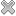# Courses / Module

Toggle Print

##MATHEMATICS II

Module code: EE112FZ
Credits: 5
Semester: 2
Department: INTERNATIONAL ENGINEERING COLLEGE
International:Overview

Indicative Syllabus • Introduction, motivation and scope • The Laplace Transform • Basic Laplace Transform • Inverse Laplace Transform • Solving first-order ODEs using the Laplace Transform. • Vector algebra • Vectors in coordinate independent form and in 2-Space, 3-Space and n-Space. • Addition, multiplication of vectors by a scalar. • Basic properties of vectors, subtraction and scalar (dot) and vector (cross) product of vectors. • Rules of Vector algebra. • Unit vectors i,j,k. • Inner (Dot) product in n-space and Vector (Cross) product in 3space. • Orthogonality of vectors. • Applications of inner and vector products. • Vector equation of a line in 3-space, Equation of plane in 3-space. • Triple scalar product, Triple vector product. • Time derivative of a vector function. • Vector equation of a curve in parametric form in 2 and 3 space. • Arc length, unit tangent, unit principal normal vectors. Curvature. • Matrix algebra • Overview of Matrix algebra and its usefulness in solving systems of linear equations. • Matrix addition, subtraction and multiplication. • Matrix properties – transpose, symmetric, rank • Solving linear systems of equations using Gaussian and Gauss-Jordan elimination. • Special matrices such as the identity matrix, the zero matrix, and orthogonal matrices. • Solving linear systems using the inverse of a matrix. Properties of the inverse matrix. • Calculating the inverse matrix using row operations. • Definition of the determinant and discussion of its properties. • Calculating the inverse matrix using the method of cofactors. • Solving linear systems using the inverse matrix and Cramer’s rule. • Eigenvalues and eigenvectors. • Diagonalization of matrices. Cayley-Hamilton theorem. • Linear Independence, basis and dimension, with connection to row operations and determinant.Learning OutcomesTeaching & Learning methodsAssessmentRepeat optionsTimetable